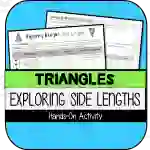Math > Geometry | Grade 7 | Activities

# Exploring Triangle Inequality Theorem (Side Lengths) HANDS-ON ACTIVITYAttributes
Subject

Geometry

Types

Activities

File

PDF

Editable
NoThis resource was created to PARTIALLY meet the requirements for the 7th Grade Math Geometry Standard below:

CCSS.MATH.CONTENT.7.G.A.2

Draw (freehand, with ruler and protractor, and with technology) geometric shapes with given conditions. Focus on constructing triangles from three measures of angles or sides, noticing when the conditions determine a unique triangle, more than one triangle, or no triangle.

## What's Included

This resource contains the following items:

1) A side-length manipulative activity where students trial-and-error different combinations of side lengths to determine a rule for side length requirements of triangles.

2) Brief summary notes and practice problems